Ex 7.5

Chapter 7 Class 6 Fractions
Serial order wise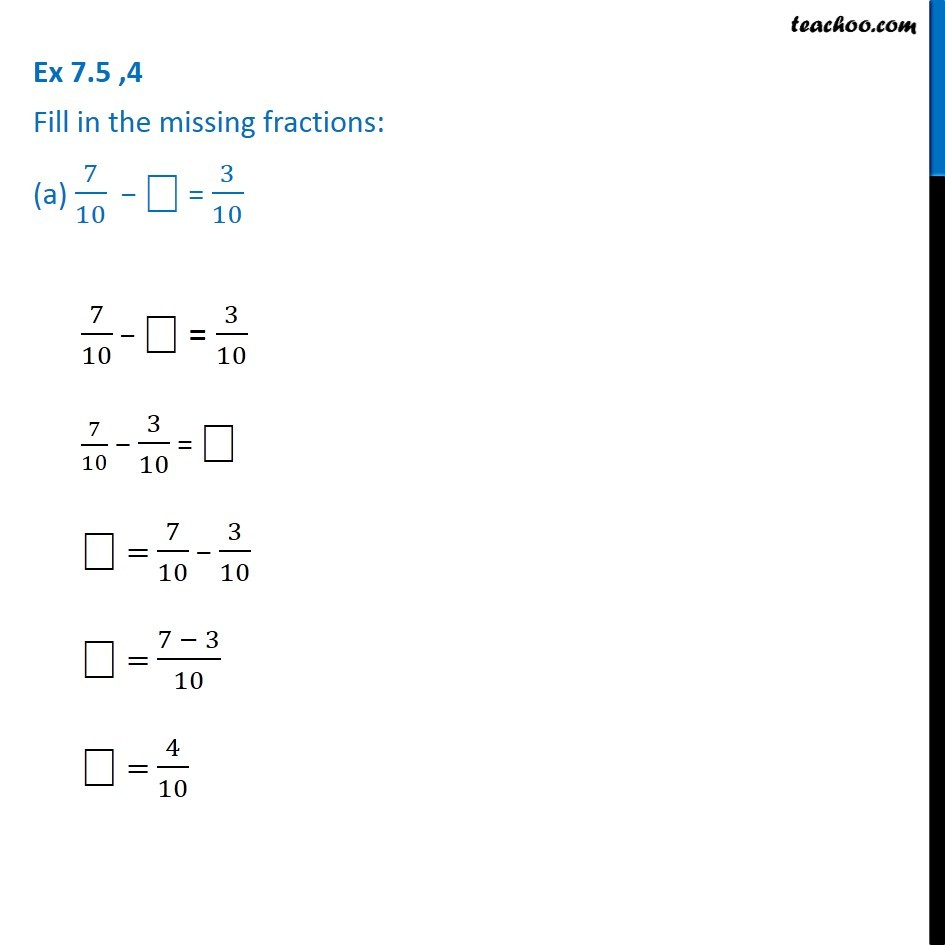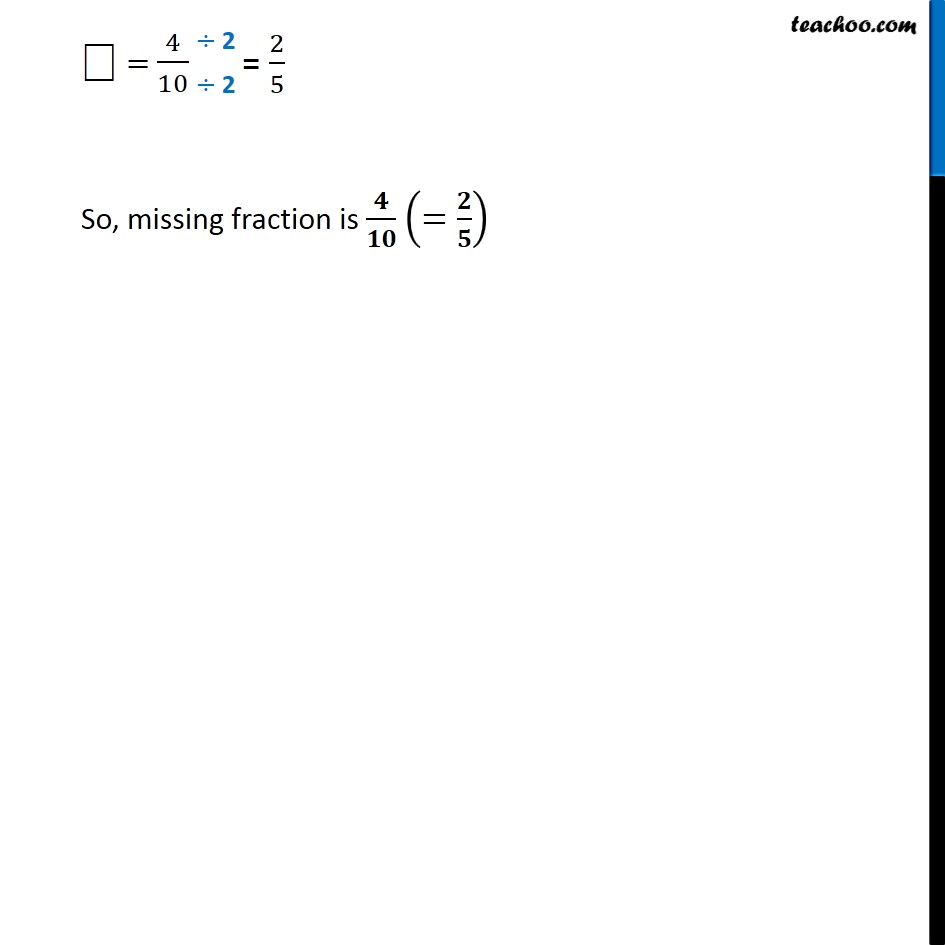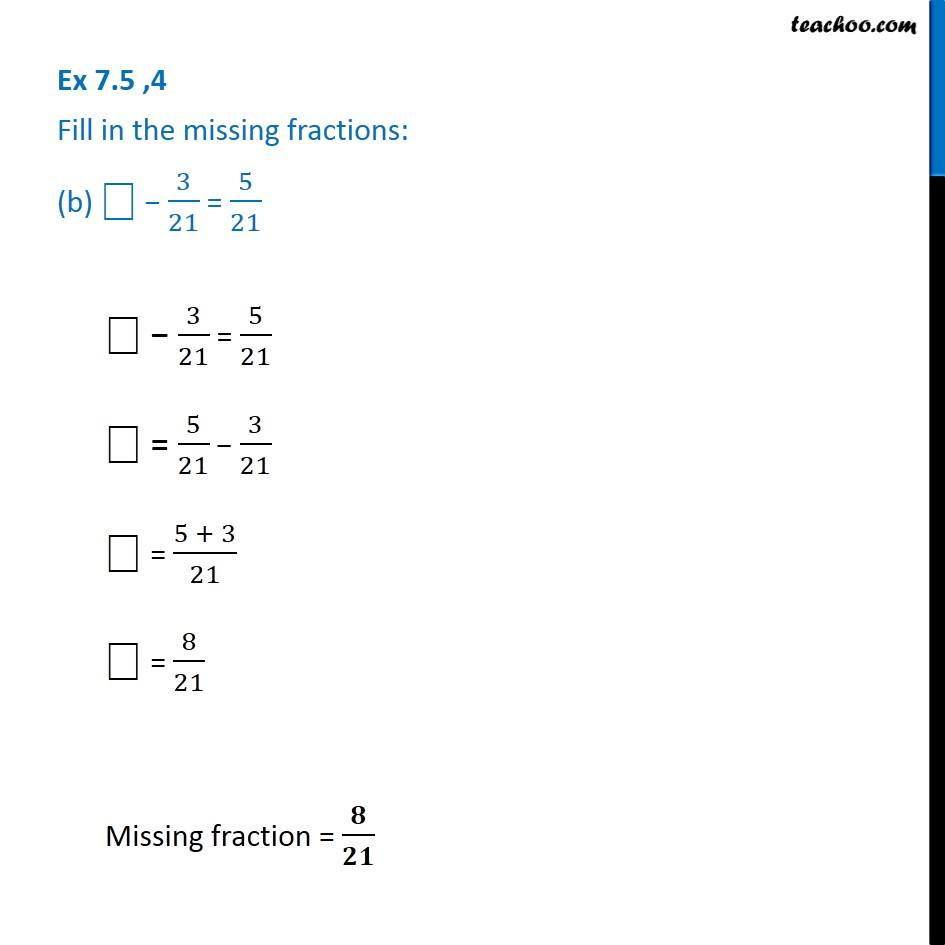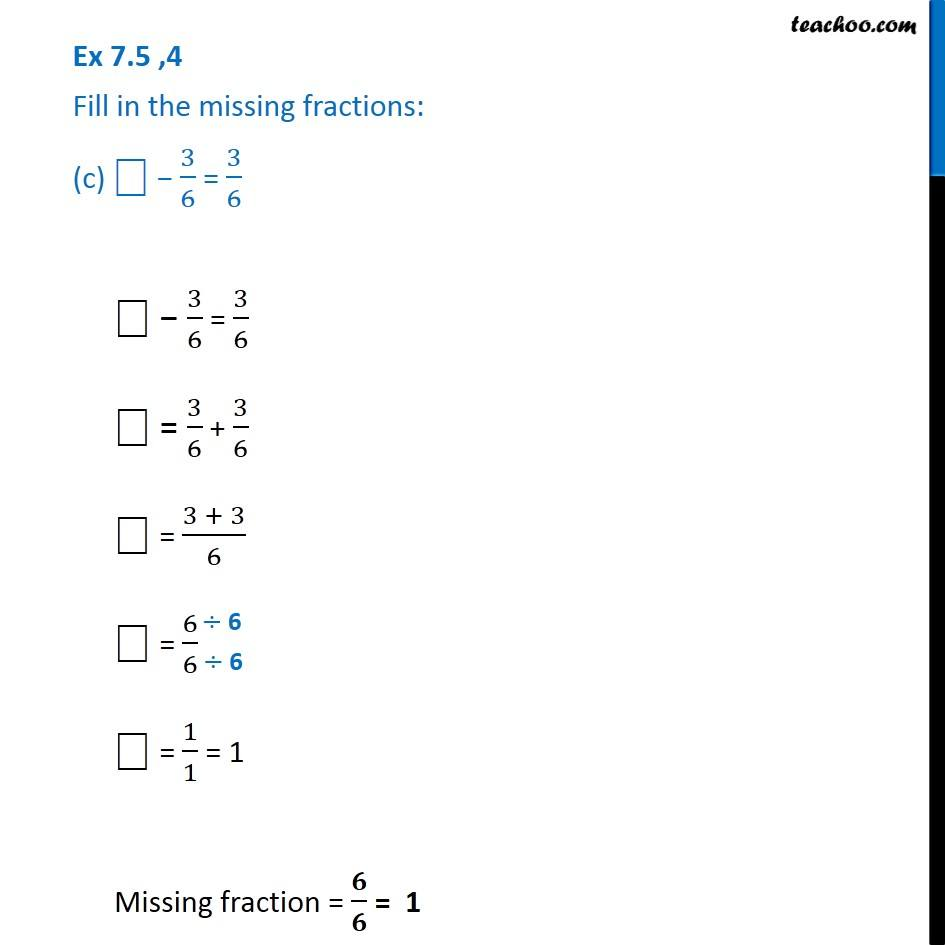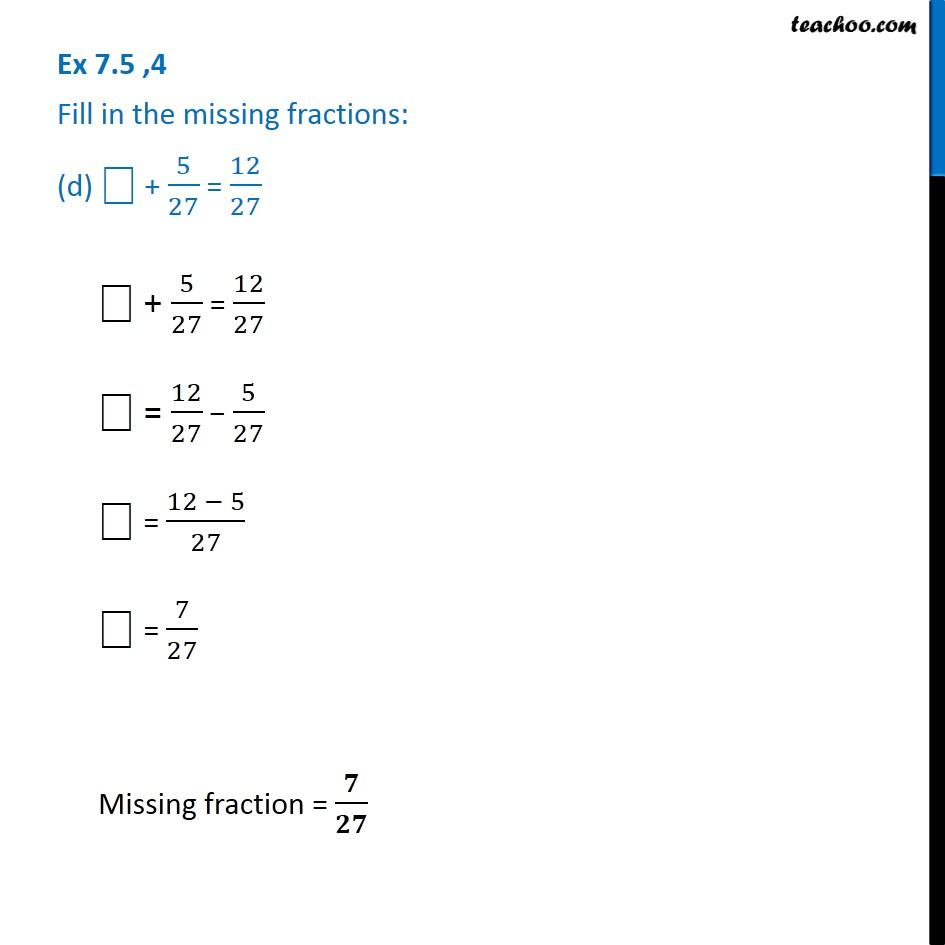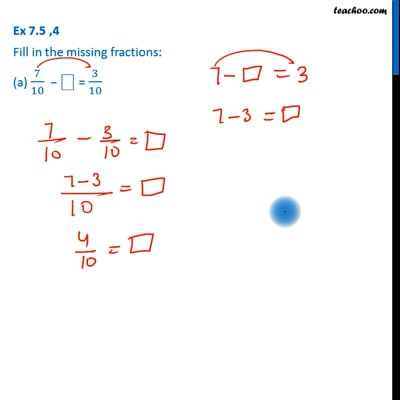This video is only available for Teachoo black users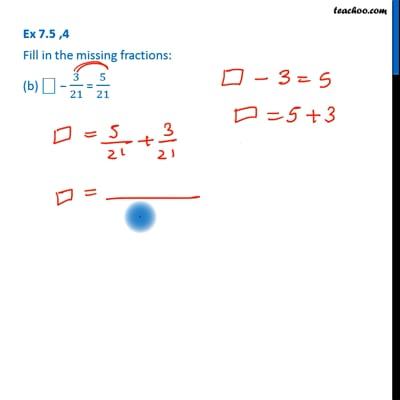This video is only available for Teachoo black users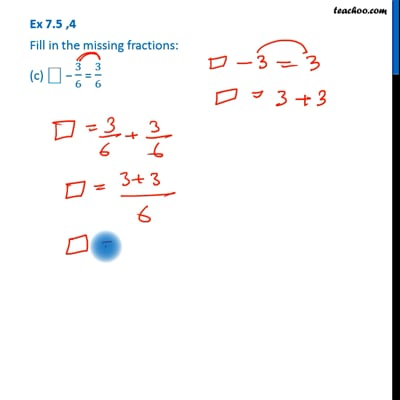This video is only available for Teachoo black users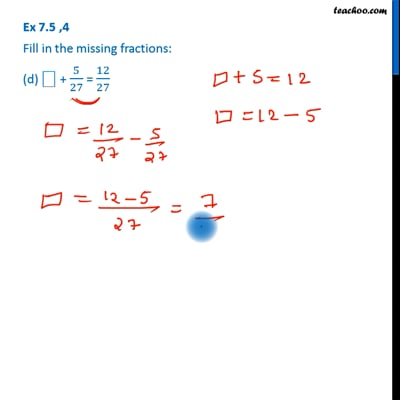This video is only available for Teachoo black users

Get live Maths 1-on-1 Classs - Class 6 to 12

### Transcript

Ex 7.5 ,4 Fill in the missing fractions: (a) 7/10 − ⎕ = 3/107/10 − ⎕ = 3/10 7/10 − 3/10 = ⎕ ⎕ = 7/10 − 3/10 ⎕ = (7 − 3)/10 ⎕ = 4/10 ⎕ = 4/10 So, missing fraction is 𝟒/𝟏𝟎 (=𝟐/𝟓) Ex 7.5 ,4 Fill in the missing fractions: (b) ⎕ − 3/21 = 5/21⎕ − 3/21 = 5/21 ⎕ = 5/21 − 3/21 ⎕ = (5 + 3)/21 ⎕ = 8/21 Missing fraction = 𝟖/𝟐𝟏 Ex 7.5 ,4 Fill in the missing fractions: (c) ⎕ − 3/6 = 3/6 ⎕ − 3/6 = 3/6 ⎕ = 3/6 + 3/6 ⎕ = (3 + 3)/6 ⎕ = 6/6 ⎕ = 1/1 = 1 Missing fraction = 𝟔/𝟔 = 1 Ex 7.5 ,4 Fill in the missing fractions: (d) ⎕ + 5/27 = 12/27 ⎕ + 5/27 = 12/27 ⎕ = 12/27 − 5/27 ⎕ = (12 − 5)/27 ⎕ = 7/27 Missing fraction = 𝟕/𝟐𝟕